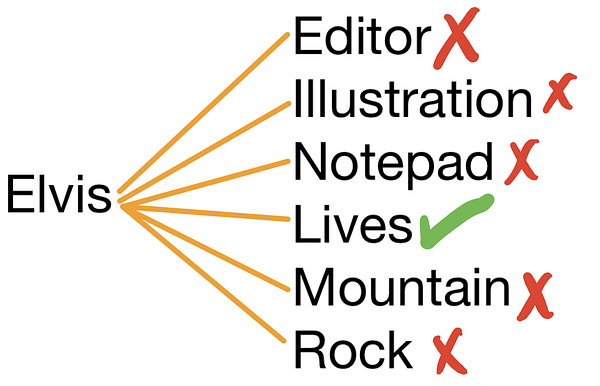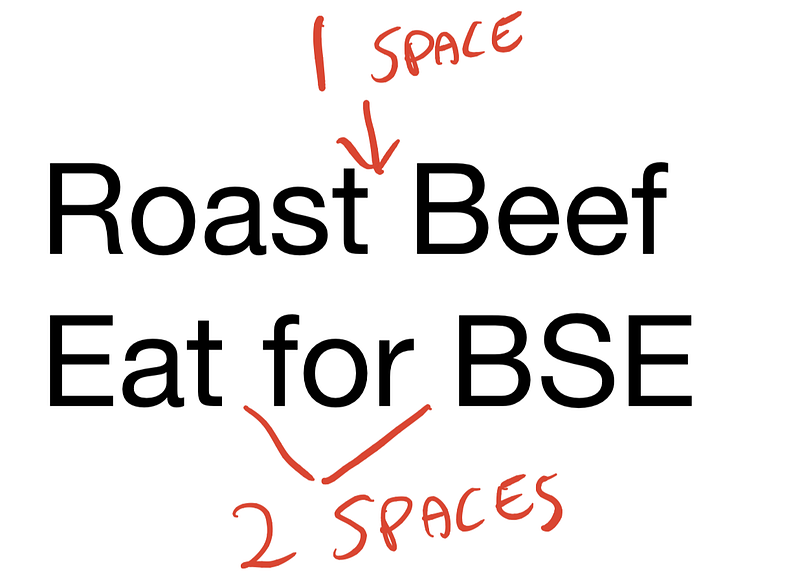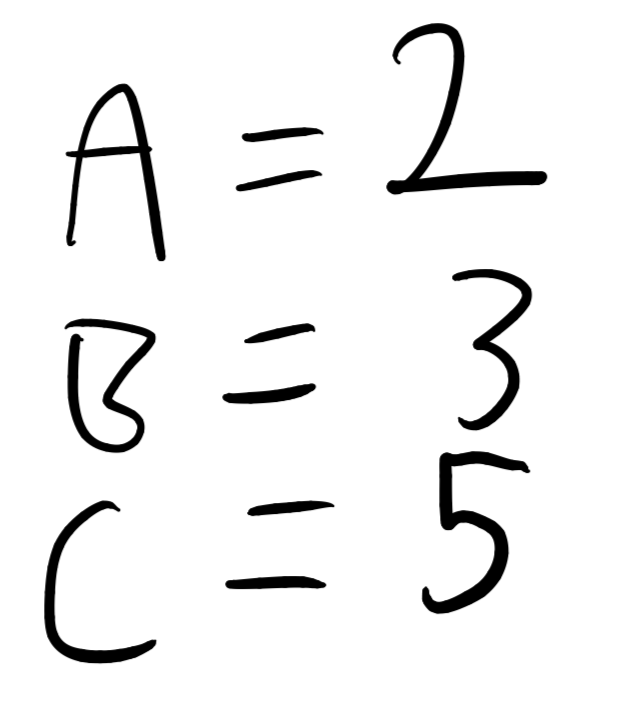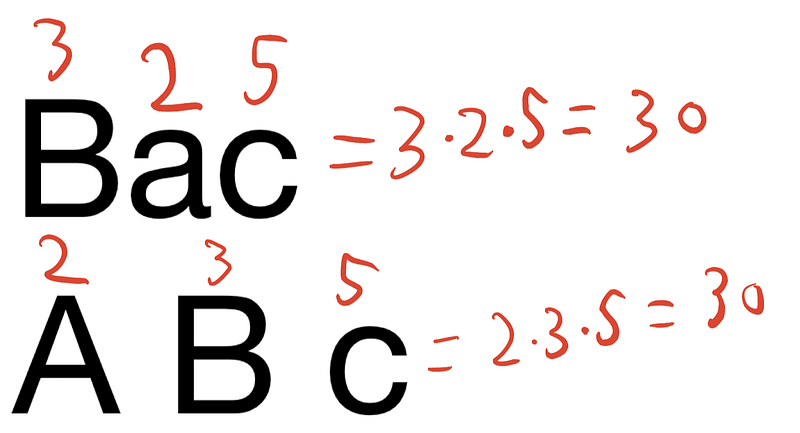Finding anagrams of words does not look like a difficult problem but has an interesting solution.

An anagram is a word or sentence that can be transformed into another word or sentence. Elvis has all the same letters as Lives, so Elvis is an anagram of Lives.The way most people would immediately solve this problem would be to take a word, go through every word in the dictionary and see if the combinations of letters match exactly.

The way to do this will use a multiset. *Sets *are like arrays where the order doesn’t matter and repetitions aren’t allowed. With an array, [a, b] is not the same as [b, a]. But with a set, (a, b) is the same as (b, a).

Set’s don’t allow repetitions. So (a, a, a, a, a, b) is the same as (b, a) — because the first set would be turned into (a, b).

A *multiset *is a set which allows repetitions but the order doesn’t matter. For this example, let’s start small.

With our example, we have a list called a dictionary where each item in that dictionary is a word. We want to find out which of these words are anagrams of “Elvis”, so what we do is for loop through the dictionary like so:

Note: this isn’t how multisets work in Python, but I’m mainly using this to illustrate a point. You can find out how multisets work here.You, probably. You’re right. This is really slow. It would work, but we have 2 problems. The first problem is that capitalisation won’t make the multisets equal. The second problem is that more spaces can appear in the outputted sentence than in the original word.As an example, “roast beef” is an anagram of “eat for BSE”.

Given 2 sentences such as “roast beef” and “eat for BSE”, if we turned these into multisets they wouldn’t be equal due to the differing amount of spaces. There’s another way we can calculate if 2 sentences are anagrams of each other. The fundamental theorem of arithmetic states:

Every integer either is a prime number itself or can be represented as the product of prime numbers and that, moreover, this representation is unique, the order of the factors.

Prime numbers are numbers which only have 2 factors — the number itself and 1.

If we assign each letter in the alphabet to a prime number, like so:And so on, then compute the product of these numbers this number is unique — because of the fundamental theorem of arithmetic.

That means that for a multiset of letters, the product of prime numbers for each letter in that multiset is unique. If two words or sentences have the same number, these two words or sentences are anagrams of each other. Let’s see a quick example, is “BAC” an anagram of “A Bc”?Determining which words are anagrams of other words would be as simple as creating a dictionary of {Word: Prime factorisation} and then grouping all the prime factorisation up.

Now, given 2 sentences we can easily tell if they are anagrams of each other.

## We can go faster#

This prime factorisation trick is interesting, but it doesn’t scale very well. Prime numbers grow exponentially, so the longer the message the larger the numbers - meaning the slower it becomes.

A much quicker way to check for anagrams is to sort the message, ascending from A to B while removing all punctuation / spaces. There are many ways to sort data, for example Timsort is a sorting algorithm.

Once you’ve sorted the two sentences / words you want to compare, if each list is equal to eachother then they are anagrams.

Hey 👋 Want to subscribe to my blog and stay up to date with posts similar to this one? Subscribe to my email list below. I won’t spam you. I will only send you posts similar to this one 😊✨

``````#mc_embed_signup{background:#fff; clear:left; font:14px Helvetica,Arial,sans-serif; }
/* Add your own Mailchimp form style overrides in your site stylesheet or in this style block.
We recommend moving this block and the preceding CSS link to the HEAD of your HTML file. */

#mc-embedded-subscribe-form input[type=checkbox]{display: inline; width: auto;margin-right: 10px;}
#mergeRow-gdpr {margin-top: 20px;}
#mergeRow-gdpr fieldset label {font-weight: normal;}